Question

# How many grams of Na2SO4 should be added to 228 g of water to make a...

How many grams of Na2SO4 should be added to 228 g of water to make a solution with a colligative molality of 0.530 m?

____g

Na2SO4 is a strong salt : Na2SO4 = 2 Na+ + SO42- for which i = Vant'Hoff factor = 2 + 1 = 3

0.530 = moles ions/ 0.228 Kg
moles ions =0.530×0.228=0.1208

moles Na2SO4 = 0.1208/3=0.0402

mass Na2SO4 = 0.0402 x 142 g/mol=5.708 g

Thank you

#### Earn Coins

Coins can be redeemed for fabulous gifts.

Similar Homework Help Questions
• ### How many grams of water should be added to 1.75 grams of fructose to give a...

How many grams of water should be added to 1.75 grams of fructose to give a 0.126 molal solution? (MW of fructose = 180.16 g/mol)

• ### How many grams of magnesium sulfate should be added to 425 mL of de-ionized water to...

How many grams of magnesium sulfate should be added to 425 mL of de-ionized water to make a 0.075 M solution?

• ### How many grams of MgCl2 (molar mass = 95.2 g/mol) should be added to 300 grams...

How many grams of MgCl2 (molar mass = 95.2 g/mol) should be added to 300 grams of water to give a solution that freezes at -3.00°C? Kf of H2O is 1.86°C/m.

• ### How many grams of NaCl would need to be added to 4001 g of water to...

How many grams of NaCl would need to be added to 4001 g of water to increase the boiling temperature of the solution by 1.500 °C? (Kb for water is 0.5100 °C/m)?

• ### 17) How many grams of water are in 250.0 g of a 5% by mass solution?...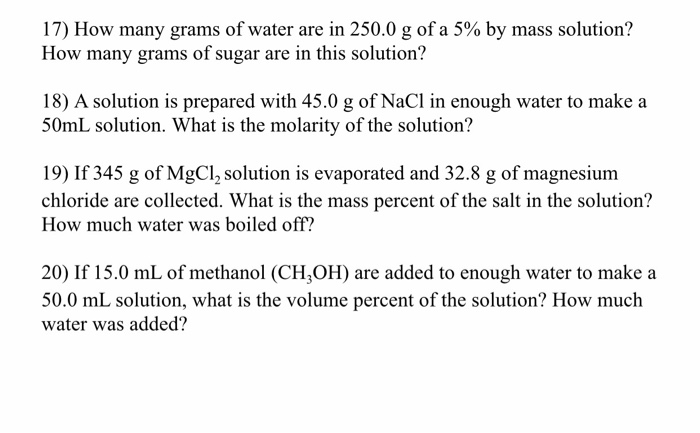17) How many grams of water are in 250.0 g of a 5% by mass solution? How many grams of sugar are in this solution? 18) A solution is prepared with 45.0 g of NaCl in enough water to make a 50mL solution. What is the molarity of the solution? 19) If 345 g of MgCl, solution is evaporated and 32.8 g of magnesium chloride are collected. What is the mass percent of the salt in the solution? How much...

• ### How many grams of CaCl2 are needed to make 5926 g of a solution that is...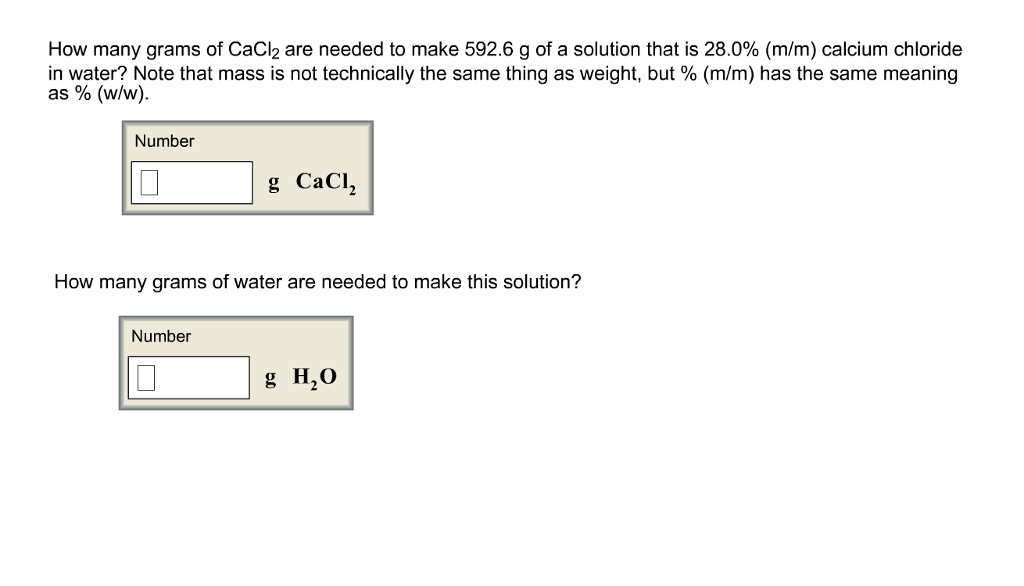How many grams of CaCl2 are needed to make 5926 g of a solution that is 28.0% (m/m) calcium chloride in water? Note that mass is not technically the same thing as weight, but % (m/m) has the same meaning as % (w/w). Number g CaCl How many grams of water are needed to make this solution? Number g H20

• ### How many grams of CaCl, are needed to make 974.0 g of a solution that is...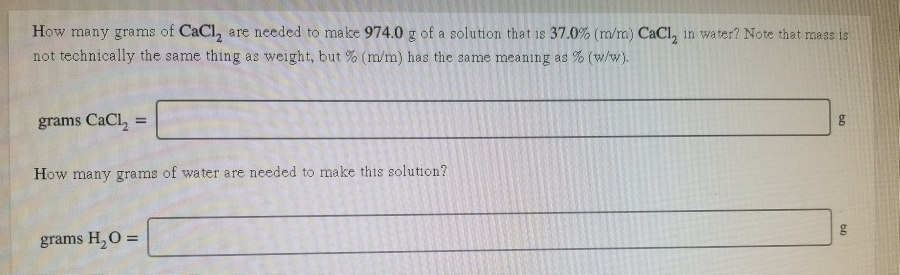How many grams of CaCl, are needed to make 974.0 g of a solution that is 37.0% (m/m) CaCl, in water? Note that mass is not technically the same thing as weight, but % (m/m) has the same meaning as % (w/w). grams CaCl2 = How many grams of water are needed to make this solution? grams H,O=

• ### How many grams of CaCl, are needed to make 741.8 g of a solution that is...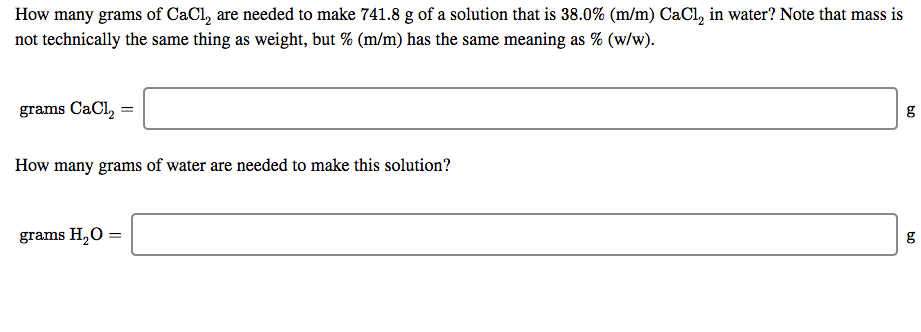How many grams of CaCl, are needed to make 741.8 g of a solution that is 38.0% (m/m) CaCl, in water? Note that mass is not technically the same thing as weight, but % (m/m) has the same meaning as % (w/w). grams CaCl, = How many grams of water are needed to make this solution? grams H,0 =

• ### PART A How many grams of ethanol, CH3CH2OH, should you dissolve in water to make 0.400...

PART A How many grams of ethanol, CH3CH2OH, should you dissolve in water to make 0.400 L of vodka (which is an aqueous solution that is 6.86 M ethanol)? Express your answer with the appropriate units. PART B Using the density of ethanol (0.789 g/mL), calculate the volume of ethanol you need to make 0.400 L of vodka. Express your answer with the appropriate units.

• ### How many grams of solid ammonium chloride should be added to 1.00 L of a 0.281...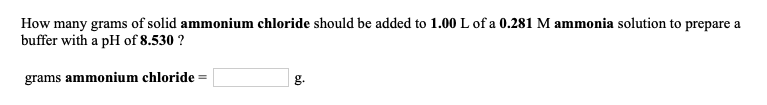How many grams of solid ammonium chloride should be added to 1.00 L of a 0.281 M ammonia solution to prepare a buffer with a pH of 8.530 ? grams ammonium chloride = g. How many grams of solid sodium acetate should be added to 0.500 L of a 9.49x10-2 M acetic acid solution to prepare a buffer with a pH of 5.309? grams sodium acetate =